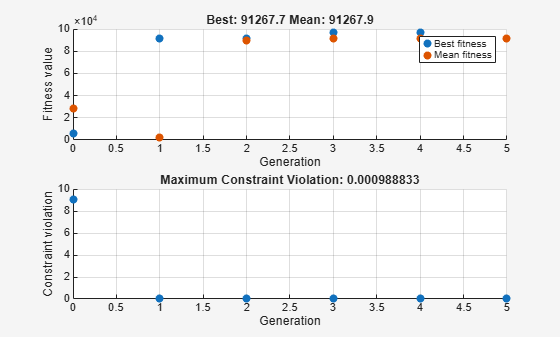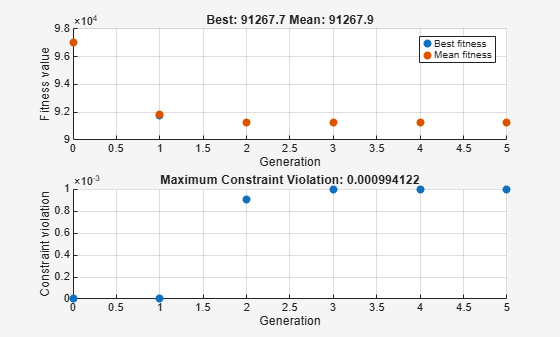# Constrained Minimization Using the Genetic Algorithm

This example shows how to minimize an objective function subject to nonlinear inequality constraints and bounds using the Genetic Algorithm.

### Constrained Minimization Problem

For this problem, the objective function to minimize is a simple function of a 2-D variable `x`.

`simple_objective(x) = (4 - 2.1*x(1)^2 + x(1)^4/3)*x(1)^2 + x(1)*x(2) + (-4 + 4*x(2)^2)*x(2)^2;`

This function is known as "cam," as described in L.C.W. Dixon and G.P. Szego .

Additionally, the problem has nonlinear constraints and bounds.

``` x(1)*x(2) + x(1) - x(2) + 1.5 <= 0 (nonlinear constraint) 10 - x(1)*x(2) <= 0 (nonlinear constraint) 0 <= x(1) <= 1 (bound) 0 <= x(2) <= 13 (bound) ```

### Code the Fitness Function

Create a MATLAB file named `simple_objective.m` containing the following code:

`type simple_objective`
```function y = simple_objective(x) %SIMPLE_OBJECTIVE Objective function for PATTERNSEARCH solver % Copyright 2004 The MathWorks, Inc. x1 = x(1); x2 = x(2); y = (4-2.1.*x1.^2+x1.^4./3).*x1.^2+x1.*x2+(-4+4.*x2.^2).*x2.^2; ```

Solvers such as `ga` accept a single input `x`, where `x` has as many elements as the number of variables in the problem. The objective function computes the scalar value of the objective function and returns it in its single output argument `y`.

### Code the Constraint Function

Create a MATLAB file named `simple_constraint.m` containing the following code:

`type simple_constraint`
```function [c, ceq] = simple_constraint(x) %SIMPLE_CONSTRAINT Nonlinear inequality constraints. % Copyright 2005-2007 The MathWorks, Inc. c = [1.5 + x(1)*x(2) + x(1) - x(2); -x(1)*x(2) + 10]; % No nonlinear equality constraints: ceq = []; ```

The constraint function computes the values of all the inequality and equality constraints and returns the vectors `c` and `ceq`, respectively. The value of `c` represents nonlinear inequality constraints that the solver attempts to make less than or equal to zero. The value of `ceq` represents nonlinear equality constraints that the solver attempts to make equal to zero. This example has no nonlinear equality constraints, so `ceq = []`. For details, see Nonlinear Constraints.

### Minimizing Using `ga`

Specify the objective function as a function handle.

`ObjectiveFunction = @simple_objective;`

Specify the problem bounds.

```lb = [0 0]; % Lower bounds ub = [1 13]; % Upper bounds```

Specify the nonlinear constraint function as a function handle.

`ConstraintFunction = @simple_constraint;`

Specify the number of problem variables.

`nvars = 2;`

Call the solver, requesting the optimal point `x` and the function value at the optimal point `fval`.

```rng default % For reproducibility [x,fval] = ga(ObjectiveFunction,nvars,[],[],[],[],lb,ub,ConstraintFunction)```
```Optimization terminated: average change in the fitness value less than options.FunctionTolerance and constraint violation is less than options.ConstraintTolerance. ```
```x = 1×2 0.8122 12.3103 ```
```fval = 9.1268e+04 ```

To observe the solver's progress, specify options that select two plot functions. The plot function `gaplotbestf` plots the best objective function value at every iteration, and the plot function `gaplotmaxconstr` plots the maximum constraint violation at every iteration. Set these two plot functions in a cell array. Also, display information about the solver's progress in the Command Window by setting the `Display` option to `'iter'`.

```options = optimoptions("ga",'PlotFcn',{@gaplotbestf,@gaplotmaxconstr}, ... 'Display','iter');```

Run the solver, including the `options` argument.

```[x,fval] = ga(ObjectiveFunction,nvars,[],[],[],[],lb,ub, ... ConstraintFunction,options)```
```Single objective optimization: 2 Variable(s) 2 Nonlinear inequality constraint(s) Options: CreationFcn: @gacreationuniform CrossoverFcn: @crossoverscattered SelectionFcn: @selectionstochunif MutationFcn: @mutationadaptfeasible Best Max Stall Generation Func-count f(x) Constraint Generations 1 2520 91342.3 0 0 2 4982 91324.1 4.605e-05 0 3 7914 97166.5 0 0 4 16157 96997.8 0 0 5 20675 91267.2 0.0009994 0 Optimization terminated: average change in the fitness value less than options.FunctionTolerance and constraint violation is less than options.ConstraintTolerance. ``````x = 1×2 0.8123 12.3103 ```
```fval = 9.1267e+04 ```

With iterative display, that `ga` provides details about the problem type and the creation, crossover, mutation, and selection operators.

Nonlinear constraints cause `ga` to solve many subproblems at each iteration. As shown in both the plots and the iterative display, the solution process has few iterations. However, the `Func-count` column in the iterative display shows many function evaluations per iteration.

The `ga` solver handles linear constraints and bounds differently from nonlinear constraints. All the linear constraints and bounds are satisfied throughout the optimization. However, `ga` may not satisfy all the nonlinear constraints at every generation. If `ga` converges to a solution, the nonlinear constraints will be satisfied at that solution.

`ga` uses the mutation and crossover functions to produce new individuals at every generation. The way the `ga` satisfies the linear and bound constraints is to use mutation and crossover functions that only generate feasible points. For example, in the previous call to `ga`, the default mutation function (for unconstrained problems) `mutationgaussian` does not satisfy the linear constraints and so `ga` uses the `mutationadaptfeasible` function instead by default. If you provide a custom mutation function, this custom function must only generate points that are feasible with respect to the linear and bound constraints. All the crossover functions in the toolbox generate points that satisfy the linear constraints and bounds.

However, when your problem contains integer constraints, `ga` enforces that all iterations satisfy bounds and linear constraints. This feasibility occurs for all mutation, crossover, and creation operators, to within a small tolerance.

### Provide a Start Point

To speed the solver, you can provide an initial population in the `InitialPopulationMatrix` option. `ga` uses the initial population to start its optimization. Specify a row vector or a matrix where each row represents one start point.

```X0 = [0.8 12.5]; % Start point (row vector) options.InitialPopulationMatrix = X0; [x,fval] = ga(ObjectiveFunction,nvars,[],[],[],[],lb,ub, ... ConstraintFunction,options)```
```Single objective optimization: 2 Variable(s) 2 Nonlinear inequality constraint(s) Options: CreationFcn: @gacreationuniform CrossoverFcn: @crossoverscattered SelectionFcn: @selectionstochunif MutationFcn: @mutationadaptfeasible Best Max Stall Generation Func-count f(x) Constraint Generations 1 2500 92164.1 0 0 2 4950 91289.2 0.0009729 0 3 7400 91267.4 0.0009877 0 4 9850 91267.3 0.0009912 0 5 12300 91267.3 0.0009912 1 Optimization terminated: average change in the fitness value less than options.FunctionTolerance and constraint violation is less than options.ConstraintTolerance. ``````x = 1×2 0.8122 12.3103 ```
```fval = 9.1267e+04 ```

In this case, providing a start point does not substantially change the solver progress.

### References

 Dixon, L. C. W., and G .P. Szego (eds.). Towards Global Optimisation 2. North-Holland: Elsevier Science Ltd., Amsterdam, 1978.

## SupportGet trial now